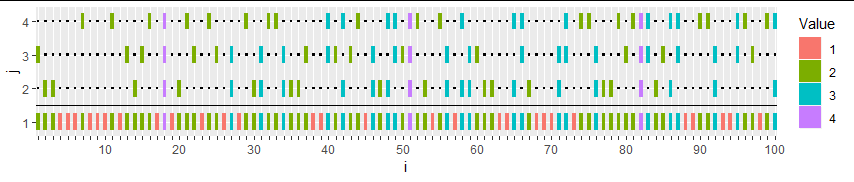Want to share your content on R-bloggers? click here if you have a blog, or here if you don't.Following an X validated question on how to simulate a multinomial with fixed average, W. Huber produced a highly elegant and efficient resolution with the compact R code

```tabulate(sample.int((k-1)*n, s-n) %% n + 1, n) + 1
```

where k is the number of classes, n the number of draws, and s equal to n times the fixed average. The R function sample.int is an alternative to sample that seems faster. Breaking the outcome of

```sample.int((k-1)*n, s-n)
```

as nonzero positions in an n x (k-1) matrix and adding a adding a row of n 1’s leads to a simulation of integers between 1 and k by counting the 1’s in each of the n columns, which is the meaning of the above picture. Where the colour code is added after counting the number of 1’s. Since there are s 1’s in this matrix, the sum is automatically equal to s. Since the s-n positions are chosen uniformly over the n x (k-1) locations, the outcome is uniform. The rest of the R code is a brutally efficient way to translate the idea into a function. (By comparison, I brute-forced the question by suggesting a basic Metropolis algorithm.)